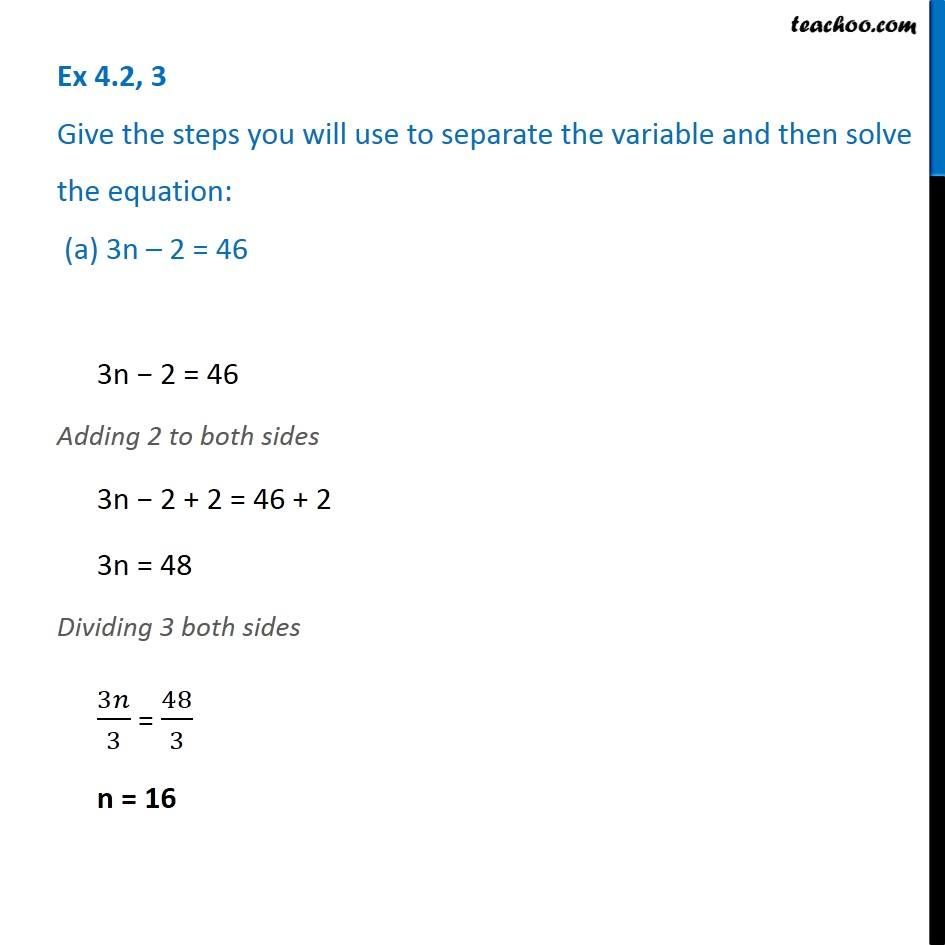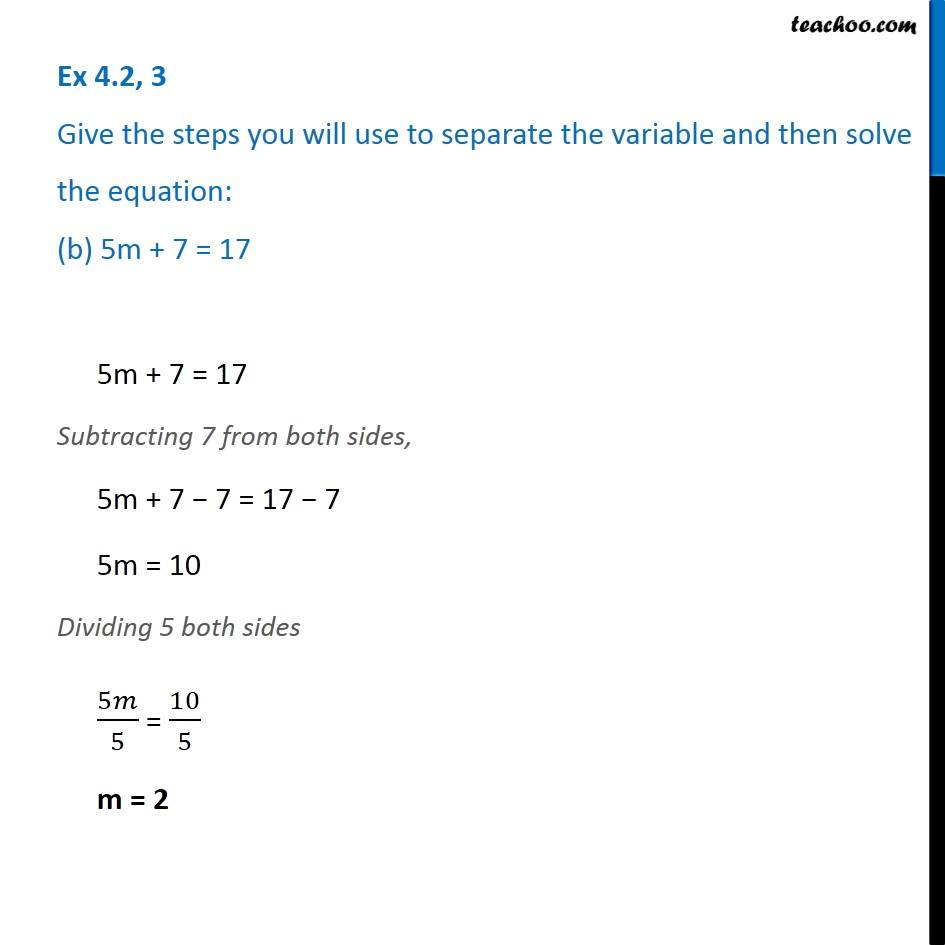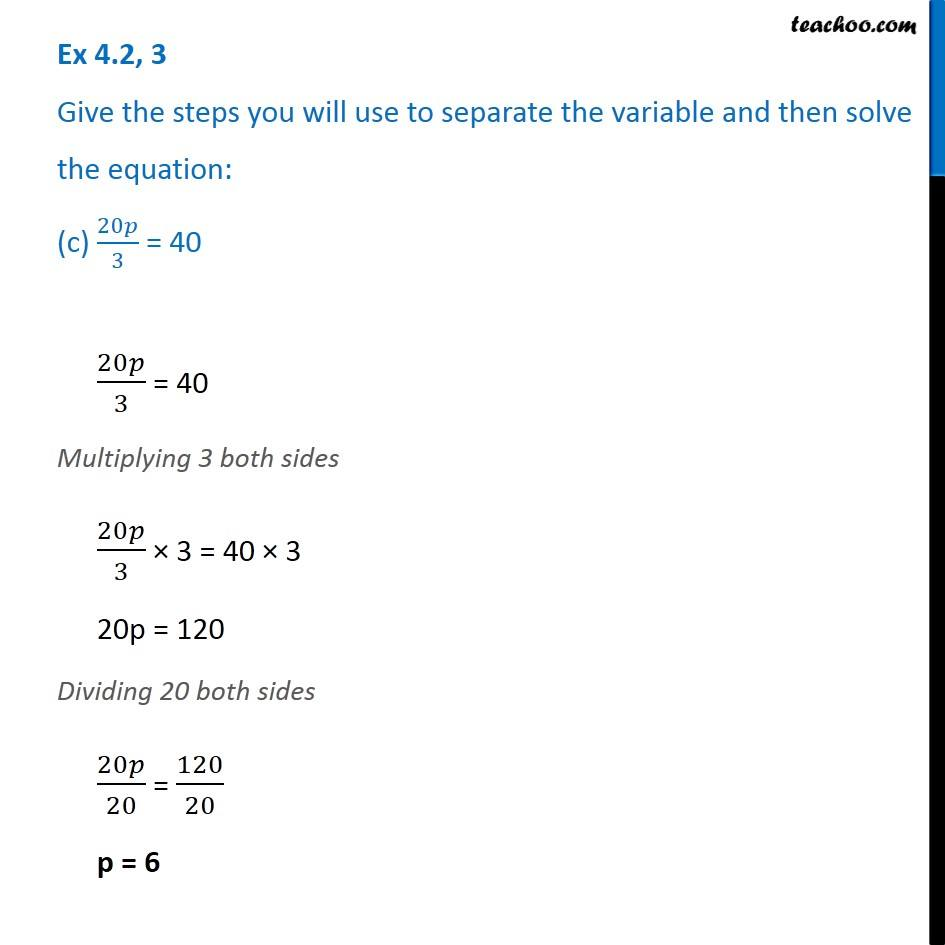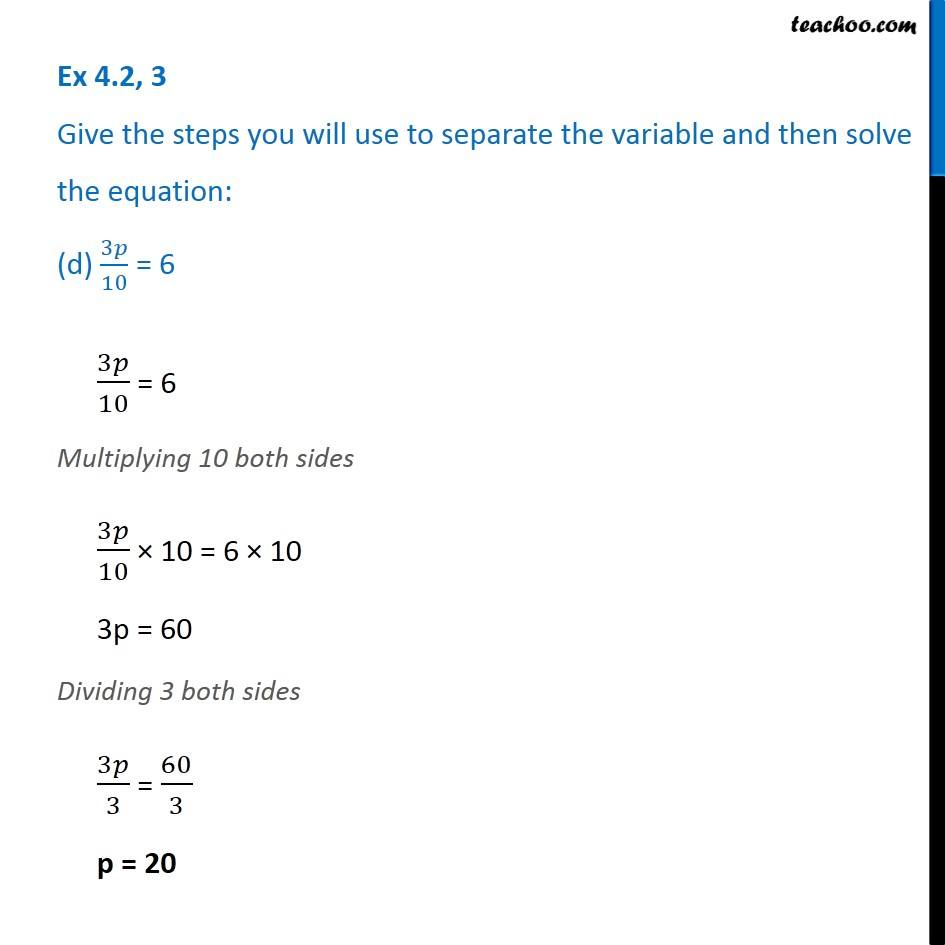1. Chapter 4 Class 7 Simple Equations
2. Concept wise
3. Solving Equations

Transcript

Ex 4.2, 3 Give the steps you will use to separate the variable and then solve the equation: (a) 3n – 2 = 46 3n − 2 = 46 Adding 2 to both sides 3n − 2 + 2 = 46 + 2 3n = 48 Dividing 3 both sides 3𝑛/3 = 48/3 n = 16 Ex 4.2, 3 Give the steps you will use to separate the variable and then solve the equation: (b) 5m + 7 = 175m + 7 = 17 Subtracting 7 from both sides, 5m + 7 − 7 = 17 − 7 5m = 10 Dividing 5 both sides 5𝑚/5 = 10/5 m = 2 Ex 4.2, 3 Give the steps you will use to separate the variable and then solve the equation: (c) 20𝑝/3 = 4020𝑝/3 = 40 Multiplying 3 both sides 20𝑝/3 × 3 = 40 × 3 20p = 120 Dividing 20 both sides 20𝑝/20 = 120/20 p = 6 Ex 4.2, 3 Give the steps you will use to separate the variable and then solve the equation: (d) 3𝑝/10 = 63𝑝/10 = 6 Multiplying 10 both sides 3𝑝/10 × 10 = 6 × 10 3p = 60 Dividing 3 both sides 3𝑝/3 = 60/3 p = 20

Solving Equations